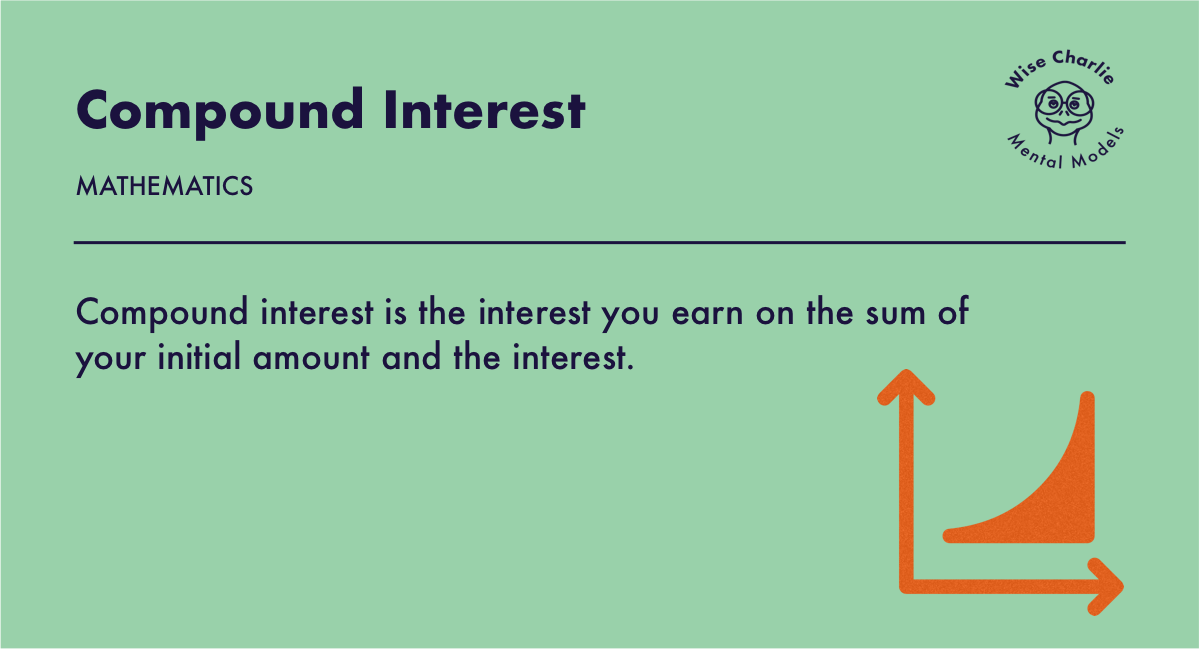# Compound InterestCompound interest is the interest you earn on the sum of your initial principal amount and the interest accumulated.

Think of it as a snowball effect. Snowball rolling down the hill, accumulating more snow and gaining in size. Snow keeps compounding as it accelerates downwards.

## Definition

Interest is the cost of borrowing or an income on a deposit. When money is lent, a simple interest is calculated on the original, principal sum. This is interest. Compound interest is calculated on the principal amount plus the accumulated interest of previous periods, and can thus be regarded as interest on interest.

It is the result of reinvesting interest, rather than paying it out, so that interest in the next period is then earned on the principal sum plus previously accumulated interest. Compound interest is standard in finance and economics.

The rate at which compound interest accrues depends on the frequency of compounding. The higher the number of compounding periods, the greater the compound interest.

## Deep Analysis

What else compounds other than money and mathematical equations?

Compound interest is well known in the field of modern finance but money is not the only thing that compounds. The power of compounding applies far beyond the realms of finance. Knowledge, relationships, marketing, sales or ideas are all compoundable. Even in sports. Score built on momentum can compound during the game.

Knowledge compounds rapidly, as you read more, the more connections you can make across different ideas and fields, leading to a prosperous snowball effect. The more you do of something, the more it reinforces.

You often get an advice to make daily improvements because it has long-term effects. That is called compounding. If you focus on improving 1% on daily basis, after a year, you'll be 37 times better than where you started. It can also have the opposite effect. As you decline 1% on a daily basis, you'll be far worse than where you started.

Franceso Balducci Pegolotti

Compound interest was once regarded as immoral and condemned by Roman law. In 1340, the Florentine merchant and politician, Franceso Balducci Pegolotti provided a table of compound interest in his book Pratica della mercatura of about 1340. It gives the interest on 100 lire, for rates ranging from 1% to 8%, for up to 20 years.

The Rule of 72

The rule of 72 calculates the approximate time over which an investment will double at a given rate of return. 72 can be divided by the interest (72/I) to find out time it would take to double the initial amount. It can only be used for annual compounding.

As an example, let's say an investment that has a 6% annual rate of return will double in 12 years. An investment with an 8% annual rate of return will thus double in nine years.

Compounding is Slow

Compounding take a long time which requires focus and dedication. Therefore, compound interest is an exponential effect rather than a linear effect. We can visualize this by drawing out a hockey stick. Initially, it starts out slow and then it exponentially grows. If you don’t interrupt it, compounding produces a fortune.

Compounding tiny excellence is what creates big excellence. So focus on compounding good habits and drain out the bad habits because focusing on bad habits can compound in the wrong direction and can turn out to be expensive.

Compound interest is the eighth wonder of the world. He who understands it, earns it … he who doesn’t … pays it. ― Albert Einstein